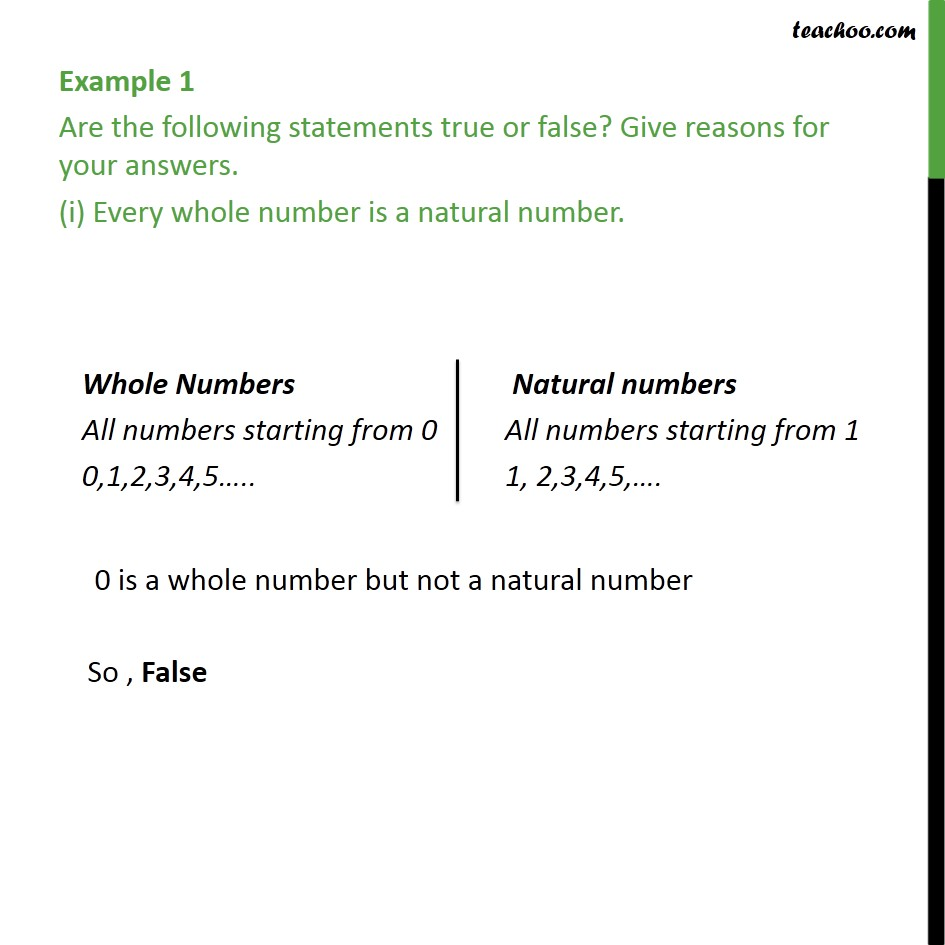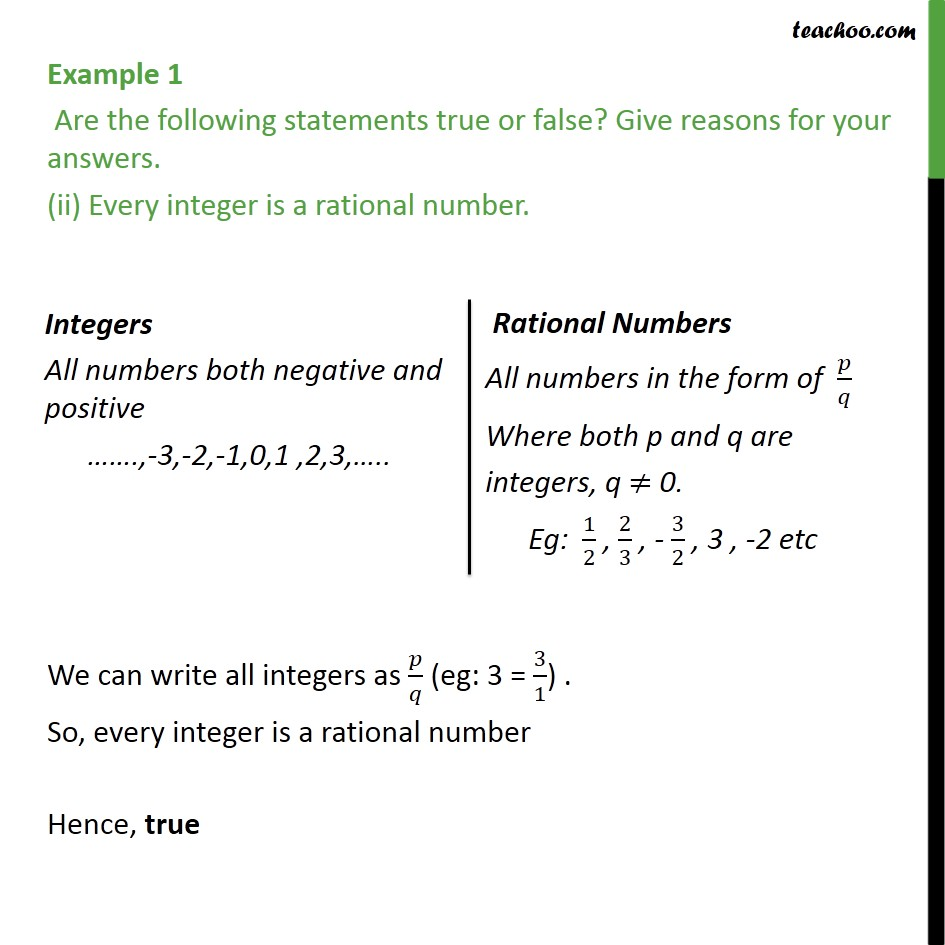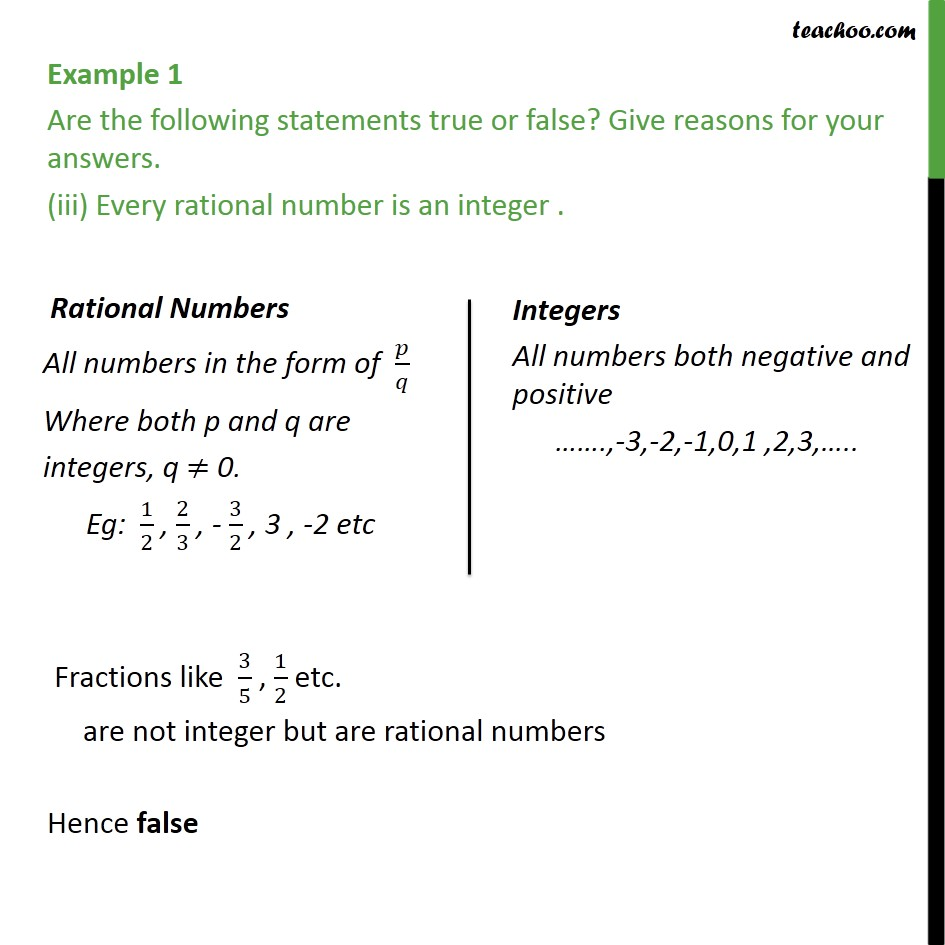Learn All Concepts of Chapter 1 Class 9 - FREE. Check - Chapter 1 Class 9 Maths1. Chapter 1 Class 9 Number Systems
2. Serial order wise
3. Examples

Transcript

Example 1 Are the following statements true or false? Give reasons for your answers. (i) Every whole number is a natural number. 0 is a whole number but not a natural number So , False Example 1 Are the following statements true or false? Give reasons for your answers. (ii) Every integer is a rational number. We can write all integers as / (eg: 3 = 3/1) . So, every integer is a rational number Hence, true Example 1 Are the following statements true or false? Give reasons for your answers. (iii) Every rational number is an integer . Fractions like 3/5 , 1/2 etc. are not integer but are rational numbers Hence false

Examples